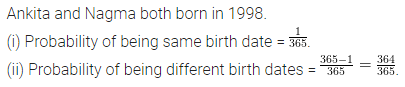## ML Aggarwal Class 8 Solutions for ICSE Maths Chapter 19 Data Handling Objective Type Questions

Mental Maths
Question 1.
Fill in the blanks:
(i) Bar graphs are ……….. representation of ungrouped data.
(ii) In a grouped frequency distribution, the difference between lower limit and upper limit of a class is called ………..
(iii) The mid point of the class interval is called ………..
(iv) Bar graphs of grouped data are called ………..
(v) The circle graphs are commonly called ………..
(vi) An experiment which has more than one possible outcomes and it is not possible to predict the outcome in advance is called ………..
(vii) The outcomes which ensures the occurrence of an event are called ………..
(viii) An event which never happens is called ………..
Solution: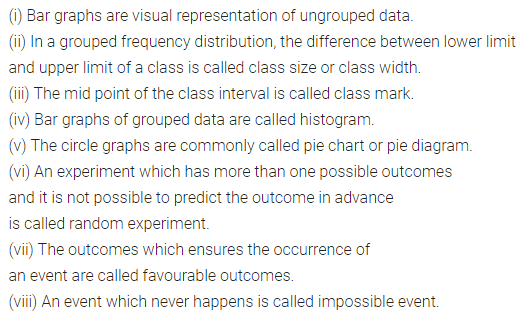Question 2.
State whether the following statements are true (T) or false (F):
(i) The data arranged in ascending or descending order of size is called data array.
(ii) The lower limit of class 10-20 is 20.
(iii) The class size of class 20-30 is 10.
(iv) The class mark of 25-35 is 30.
(v) There is no difference between bar graphs and histograms.
(vi) In histograms the breadth of a rectangle is meaningless.
(vii) In histograms, there is no gap between two adjacent rectangle.
(viii) In a pie chart, size of each sector is proportional to the value of item represented by it.
(ix) In a pie chaiangle of sector
= $$\frac{\text { value of item }}{\text { sum of values of all items }} \times 180^{\circ}$$
(x) In tossing a coin getting head or tail are equally likely events.
(xi) Probability of an event E satisfies 0 ≤ P(E) ≤ 1.
(xii) P(occurrence of an event) = P(non occurence of an event).
(xiii) Total number of outcomes when two dice are rolled togehter = 6 + 6.
Solution: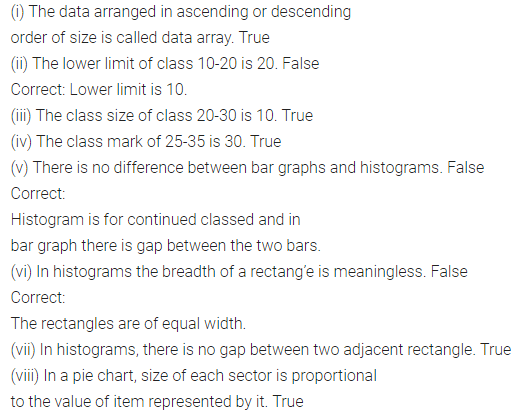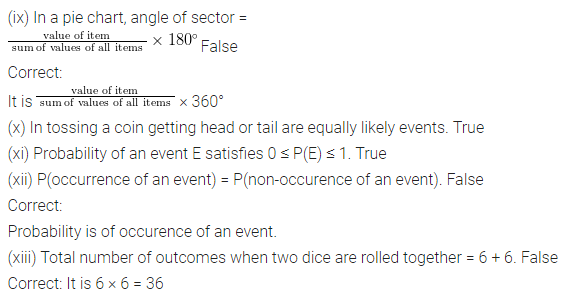Multiple Choice Questions
Study the following frequency distribution table:
The table shows the pocket money (in ?) per month of 50 students. Choose the correct answer from the given four options for questions 3 to 7;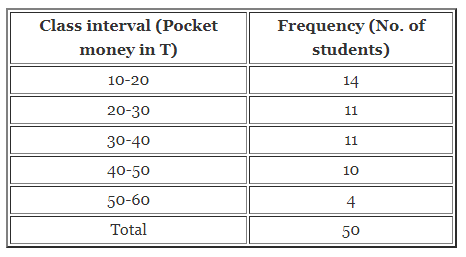Question 3.
Size of the class-intervals is
(a) 50
(b) 20
(c) 10
(d) 30
Solution: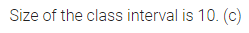Question 4.
The class having the maximum frequency is
(a) 10-20
(b) 20-30
(c) 30-40
(d) 40-50
Solution:Question 5.
The upper limit of the class having minimum frequency is
(a) 30
(b) 40
(c) 50
(d) 60
Solution: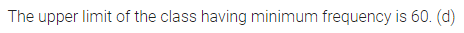Question 6.
Which two are classes having the same frequency?
(a) 10-20 and 20-30
(b) 20-30 and 30-40
(c) 30-40 and 50-60
(d) 40-50 and 50-60
Solution:Question 7.
The frequency of class whose class mark is 25 is
(a) 14
(b) 11
(c) 10
(d) 4
Solution: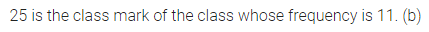The pie graph shown in the adjoining figure representing the different subjects liked by the students of class VIII. Study the pie graph carefully and choose the correct answer from the given four options for questions 8 to 11.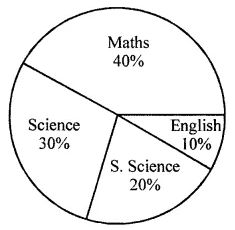Question 8.
Which subject is liked by the maximum number of students
(a) Maths
(b) Science
(c) S. Science
(d) English
Solution: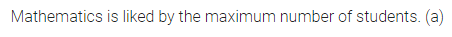Question 9.
Which subject is liked by the minimum number of students
(a) Maths
(b) Science
(c) S. Science
(d) English
Solution: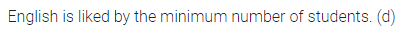Question 10.
If there are 200 students in class VIII then the number of students who like S. Science
(a) 10
(b) 20
(c) 40
(d) 80
Solution: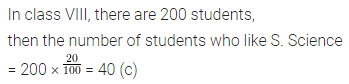Question 11.
Number of students who like Science
(a) 20
(b) 40
(c) 60
(d) 80
Solution: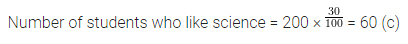Choose the correct answer from the given four options (12 to 17):
Question 12.
Probability of getting the sum as 4 when a pair of dice is rolledSolution: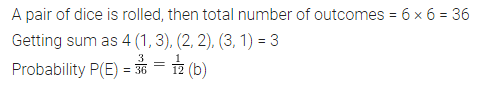Question 13.
Probability of getting exactly 2 heads when three coins are tossed together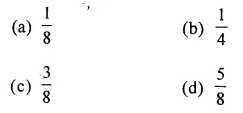Solution:Question 14.
Probability of selecting a consonant from the letters of the word ‘FATHER’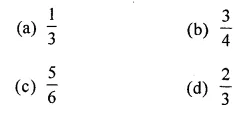Solution: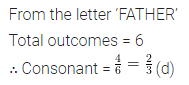Question 15.
Probability of getting more than 2 heads when a pair of coins is tossed.
(a) 1
(b) $$\frac{1}{2}$$
(c) $$\frac{1}{3}$$
(d) 0
Solution: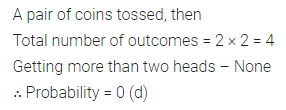Question 16.
Probability of getting a red ball from a bag containing 20 red balls
(a) 0
(b) 1
(c) $$\frac{1}{20}$$
(d) $$\frac{1}{2}$$
Solution:Question 17.
Probability of getting a non-red ball from a bag containing 4 red, 5 blue and 3 black balls is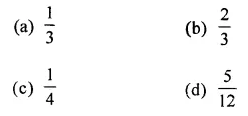Solution: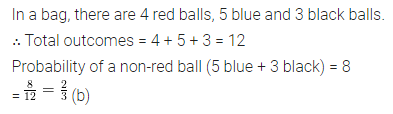Value Based Questions
Question 1.
Draw a pie chart of the data given below:
The time spent by a Class VIII student during a day.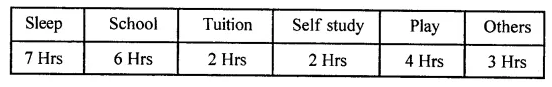Should a student of class VIII study for just 2 hours daily? Which time is considered the best time for self-study?
Solution: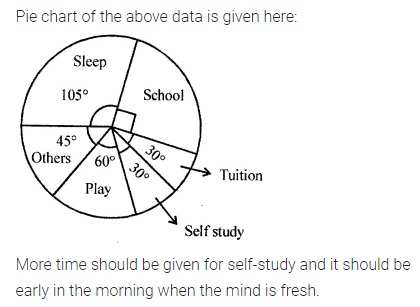Question 2.
From a bag containing 2 saffron, 3 white and 4 green balls a ball is drawn at random. Find the probability that ball drawn is
(i) Saffron
(ii) White
(iii) Green
Which are three colours in our National Flag? What values did they indicate? What values are being promoted?
Solution: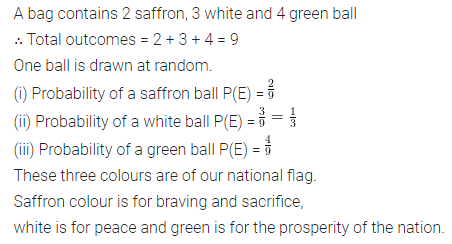Question 3.
Four defective oranges are accidentally mixed with 16 good ones. One orange is drawn at random. Find the probability that the orange drawn is good one.
What will happen if 4 bad persons are mixed with 16 good ones?
Solution:Higher Order Thinking Skills (Hots)
Question 1.
A bag contains 12 balls out of which x are black.
(i) If a ball drawn at random, what is the probability that it will be a black ball?
(ii) If 6 more black balls are put in the bag, the probability of drawing a black ball will be double than that of (i). Find the value of x.
Solution: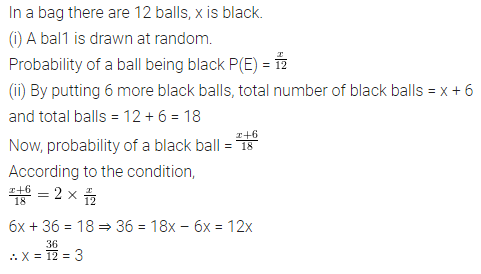Question 2.
Ankita and Nagma are friends. They were both born in 1998. What is the probability that they have
(i) same birthday?
(ii) different birthday?
Solution: